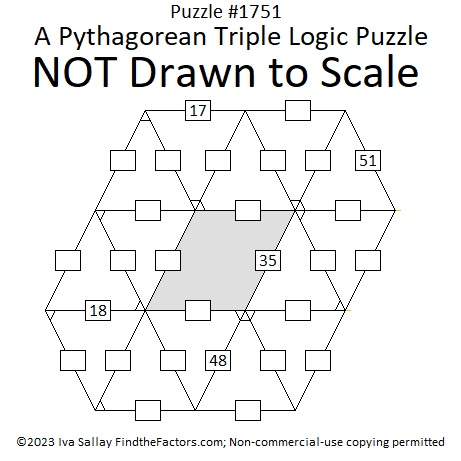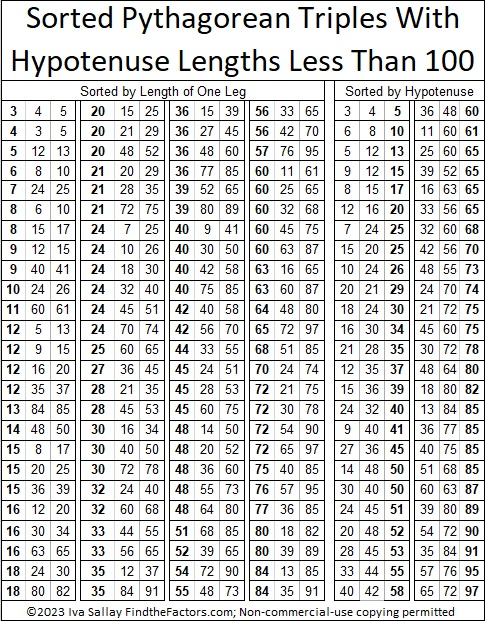# 1751 A Pythagorean Triple Puzzle

### Today’s Puzzle:

Even though they don’t look like it, the fourteen triangles in today’s puzzle are all Pythagorean triple triangles that most certainly have not been drawn to scale. The marked angle is the right angle for each of triangle. The hypotenuse of each triangle is less than 100. There is a chart under the puzzle listing all possible Pythagorean triples that could be used in the puzzle. No triple will be used more than once. Use logic to figure out what the missing side lengths are. Have fun!### Factors of 1751:

If you remember that 51 is NOT a prime number because 3 × 17 = 51, then it isn’t difficult to recognize that 1751 also must be divisible by 17.

• 1751 is a composite number.
• Prime factorization: 1751 = 17 × 103.
• 1751 has no exponents greater than 1 in its prime factorization, so √1751 cannot be simplified.
• The exponents in the prime factorization are 1 and 1. Adding one to each exponent and multiplying we get (1 + 1)(1 + 1) = 2 × 2 = 4. Therefore 1751 has exactly 4 factors.
• The factors of 1751 are outlined with their factor pair partners in the graphic below.### More About the Number 1751:

1751 is the hypotenuse of a Pythagorean triple:
824-1545-1751 which is (8-15-17) times 103.

1751 looks interesting in some other bases:
It’s 575 in base 18 because 5(18²) + 7(18) + 5(1) = 1751,
373 base 23 because 3(23²) + 7(23) + 3(1) = 1751, and
1F1 base 35 because 1(35²) + 15(35) + 1(1) = 1751.